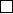5Eigenvalues and eigenvectors

IA Vectors and Matrices5.6 Canonical (Jordan normal) form
Given a matrix
A
, if its eigenvalues all have non-zero defect, then we can find
a basis in which it is diagonal. However, if some eigenvalue does have defect,
we can still put it into an almost-diagonal form. This is known as the Jordan
normal form.
Theorem. Any 2 × 2 complex matrix A is similar to exactly one of
λ
1
0
0 λ
2
,
λ 0
0 λ
,
λ 1
0 λ
Proof. For each case:
(i)
If
A
has two distinct eigenvalues, then eigenvectors are linearly independent.
Then we can use P formed from eigenvectors as its columns
(ii)
If
λ
1
=
λ
2
=
λ
and
dim E
λ
= 2, then write
E
λ
=
span{u, v}
, with
u, v
linearly independent. Now use
{u, v}
as a new basis of
C
2
and
˜
A = P
1
AP =
λ 0
0 λ
= λI
Note that since
P
1
AP
=
λI
, we have
A
=
P
(
λI
)
P
1
=
λI
. So
A
is
isotropic, i.e. the same with respect to any basis.
(iii)
If
λ
1
=
λ
2
=
λ
and
dim
(
E
λ
) = 1, then
E
λ
=
span{v}
. Now choose basis
of C
2
as {v, w}, where w C
2
\ E
λ
.
We know that
Aw C
2
. So
Aw
=
αv
+
βw
. Hence, if we change basis to
{v, w}, then
˜
A = P
1
AP =
λ α
0 β
.
However,
A
and
˜
A
both have eigenvalue
λ
with algebraic multiplicity 2.
So we must have
β
=
λ
. To make
α
= 1, let
u
= (
˜
A λI
)
w
. We know
u 6= 0 since w is not in the eigenspace. Then
(
˜
A λI)u = (
˜
A λI)
2
w =
0 α
0 0
0 α
0 0
w = 0.
So u is an eigenvector of
˜
A with eigenvalue λ.
We have u =
˜
Aw λw. So
˜
Aw = u + λw.
Change basis to {u, w}. Then A with respect to this basis is
λ 1
0 λ
.
This is a two-stage process:
P
sends basis to
{v, w}
and then matrix
Q
sends to basis
{u, w}
. So the similarity transformation is
Q
1
(
P
1
AP
)
Q
=
(P Q)
1
A(P Q).
Proposition.
(Without proof) The canonical form, or Jordan normal form,
exists for any
n × n
matrix
A
. Specifically, there exists a similarity transform
such that A is similar to a matrix to
˜
A that satisfies the following properties:
(i)
˜
A
αα
= λ
α
, i.e. the diagonal composes of the eigenvalues.
(ii)
˜
A
α,α+1
= 0 or 1.
(iii)
˜
A
ij
= 0 otherwise.
The actual theorem is actually stronger than this, and the Jordan normal
form satisfies some additional properties in addition to the above. However, we
shall not go into details, and this is left for the IB Linear Algebra course.
Example. Let
A =
3 1 1
1 3 1
2 2 0
The eigenvalues are
2
,
2
,
2 and the eigenvectors are
1
1
0
,
1
0
1
. Pick
w
=
1
0
0
. Write
u
= (
A λI
)
w
=
1 1 1
1 1 1
2 2 2
1
0
0
=
1
1
2
. Note that
Au
=
2
u
. We also have
Aw
=
u
2
w
. Form a basis
{u, w, v}
, where
v
is
another eigenvector linearly independent from u, say
1
0
1
.
Now change to this basis with
P
=
1 1 1
1 0 0
2 0 1
. Then the Jordan normal
form is P
1
AP =
2 1 0
0 2 0
0 0 2